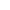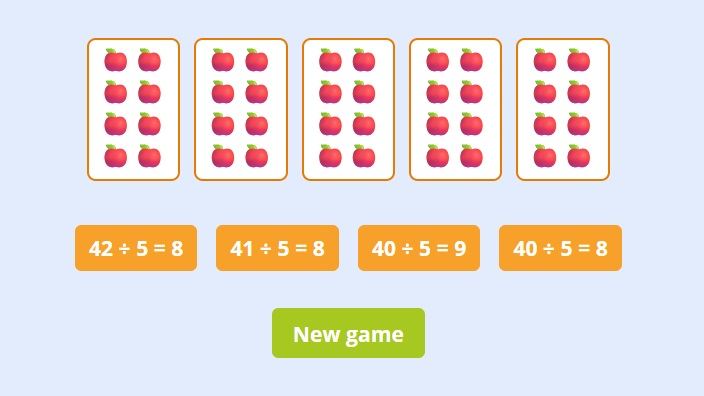﻿ Division With Pictures Games + Worksheets

Page No. 1026

# Division with pictures

Visual division with pictures games. Division as grouping method. Picture division with visuals. Learn how to perform simple division using pictures for visual representation. Division with pictures worksheets. Equal grouping in division. sharing equally. Division visual basic. Division picture problems. Equal groups division worksheets with pictures. Divide into groups. Ways to show division by sharing games. Division by grouping game. visual division worksheets.

New gameClick on the correct answer that matches the picture.

## Division with picturesWhat division problem does the picture represent? Click on the correct division expression. Press the button "New game" and play another Division with pictures game.

## Free printable division with pictures worksheets PDF

Download free printable division worksheets with pictures. Click the image bellow and generate free grouping division worksheets in PDF:

Use the pictures to work out the division problems. You can generate as many different division with pictures worksheets as you want. Find more free division worksheets here.

## Equal grouping in division

Division is a fundamental arithmetic operation that involves splitting a quantity into equal groups or parts. It is the inverse operation of multiplication. In division, one number, known as the dividend, is divided by another number, called the divisor, to obtain a quotient.

### Visual division using pictures

In this ´division by grouping game´ the total number of pictures represents the DIVIDEND, the number of groups is the DIVISOR and the QUOTIENT is number of pictures in each group.

That means in this picture:

• The DIVIDEND is 35.
• The DIVISOR is 5.
• The QUOTIENT is 7.

The correct answer is 35 ÷ 5 = 7.## Division as grouping and sharing

Division can be thought of as a process of splitting a quantity or set of objects into equal groups. This concept is often used to solve real-world problems that involve distributing items or resources evenly among a certain number of recipients.

### Divide into groups

To better understand division as splitting into groups, let's consider an example. Suppose you have 24 cookies that you want to divide equally among 6 friends. You can use division to determine how many cookies each friend will receive.

By dividing 24 cookies by 6 friends, you are essentially splitting the cookies into 6 equal groups. Each group represents the number of cookies that one friend will receive.

In other words, you would start by dividing 24 by 6. The quotient would be 4, indicating that each friend will receive 4 cookies. In this case, there is no remainder, so each friend gets exactly 4 cookies.

## Equal groups in division

This idea of splitting into equal groups is a fundamental concept in division and is often used in various scenarios, such as dividing resources among team members, sharing items equally among a group, or allocating funds among different projects.

## Division with pictures problems

We use pictures to represent the process of division by grouping because it helps illustrate the idea of dividing a quantity into equal parts and provides a clear visual representation of how the division is being carried out.

Here's an example of how pictures can aid in comprehending division by grouping:

Let's consider the previous example of dividing 24 cookies equally among 6 friends. We can draw pictures (or you can use LEGO bricks) to represent the cookies and the process of dividing them into groups:

1. Start by drawing 24 cookies or putting 24 LEGO pieces on the table.
2. Divide cookies into 6 equal parts, representing 6 friends.
3. Count the number of cookies in each group.

Each group has 4 cookies, so you can visually see that each friend will receive 4 cookies. Pictures are a helpful tool to visualize division by grouping and make the concept of division easier to understand.

## Visual division by sharing

Remember that division by grouping is just one way to understand and solve division problems. There are other methods, such as repeated subtraction, that can also be used depending on the situation and the numbers involved.

## Why to use picture model for division learning?

Division can be an abstract concept for young learners. Explaining the concept of division using pictures is simple and interactive:

• Pictures help to introduce the concept of division: Division is a way of sharing or grouping things equally. You can also use concrete examples like sharing candies or toys among friends to illustrate the idea. Watch how to explain it on YouTube here.
• Pictures are a visual aid: Visual aids can greatly enhance understanding. Draw or use objects like blocks, buttons, or counters to represent the items being divided. This will make the concept more tangible for the students.
• You can practice simple division using pictures: This game is about solving problems on equal sharing by using real-world objects to extract information. This division game requires learners to work with numbers within 50.
• Practice and repetition: This online game provide various visual division problems for students to solve. Once kids understand the principles of division, you can use division flash cards to practice simple division tasks.
• You can use pictures as visual representation of remainders: Pictures can help illustrate the concept of remainders. If the number of items being divided cannot be divided equally into the groups, there will be a remainder. For instance, if we had 25 cookies and divided them equally among 6 friends, each friend would receive 4 cookies, but there would be 1 cookie left over as a remainder. This can be represented visually by having 4 cookies in each of the 6 groups and an additional cookie remaining.

## Division sharing equally

The use of visual aids and pictures supports young students in visualizing and comprehending the abstract concept of division. It promotes active learning, engagement, and understanding, facilitating a solid foundation for their mathematical development.

Remember to use age-appropriate language, keep the explanations simple and concrete, and provide different opportunities for students to practice division problems such as division puzzles and other games. Patience and repetition are key when teaching new concepts to young students.